# RD Sharma Class 8 Solutions Chapter 18 Practical Geometry Ex 18.2

## RD Sharma Class 8 Solutions Chapter 18 Practical Geometry Ex 18.2

These Solutions are part of RD Sharma Class 8 Solutions. Here we have given RD Sharma Class 8 Solutions Chapter 18 Practical Geometry Ex 18.2

Other Exercises

Question 1.
Construct a quadrilateral ABCD in which AB = 3.8 cm, BC = 3.0 cm, AD = 2.3 cm, AC = 4.5 cm and BD = 3.8 cm.
Solution:
Steps of construction :
(i) Draw a line segment AB = 3.8 cm.
(ii) With centre A and radius 2.3 cm and with centre B and radius 3.8 cm draw arcs intersecting each other at D.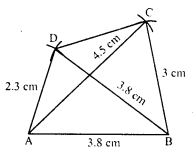(iv) Again with centre A and radius 4.5 cm and with centre B and radius 3 cm, draw arcs intersecting each other at C.
(v) Join AC and BC and also CD.
Then ABCD is the required quadrilateral.

Question 2.
Construct a quadrilateral ABCD in which BC = 7.5 cm, AC = AD = 6 cm, CD = 5 cm and BD = 10 cm.
Solution:
Steps of construction :
(i) Draw a line segment CD = 5 cm.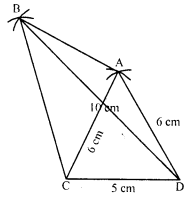(ii) With centre C and D and radius 6 cm, draw line segments intersecting each other at A.
(iv) Again with centre C and radius 7.5 cm and with centre D and radius 10 cm, draw arcs intersecting each other at B.
(v) Join CB, CA, DA, DB and AB.
Then ABCD is the required quadrilateral.

Question 3.
Construct a quadrilateral ABCD, when AB = 3 cm, CD = 3 cm, DA = 7.5 cm, AC = 8 cm and BD = 4 cm.
Solution:
Steps of construction :
This quadrilateral is not possible as
BD = 4 cm, AB = 3 cm and AD = 7.5 cm
The sum of any two sides of a triangle is greater than the third side.
But BD + AD = 4 + 3 = 7 cm

Question 4.
Construct a quadrilateral ABCD given AD = 3.5 cm, BC = 2.5 cm, CD = 4.1 cm, AC = 7.3 cm and BD = 3.2 cm.
Solution:
Steps of construction :
(i) Draw a line segment CD = 4.1 cm.
(ii) With centre C and radius 7.3 cm and with centre D and radius 3.5 cm, draw arcs intersecting each other at A.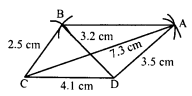(iv) Again with centre C and radius 2.5 cm and with centre D and radius 3.2 cm, draw arcs intersecting each other at B.
(v) Join CB’, and DB’ and join AB’.
Then ABCD is the required quadrilateral.

Question 5.
Construct a quadrilateral ABCD given AD = 5 cm, AB = 5.5 cm, BC = 2.5 cm, AC = 7.1 cm and BD = 8 cm.
Solution:
Steps of construction:
(i) Draw a line segment AB = 5 cm.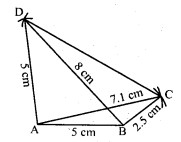(ii) With centre A and radius 7.1 cm and with centre B and radius 2.5 cm, draw arcs which intersect each other at C.
(iii) Join AC and BC.
(iv) Again with centre A and radius 5 cm and with centre B and radius 8 cm, draw arcs which intersect each other at D.
(v) Join AD and BD and CD.
Then ABCD is the required quadrilateral.

Question 6.
Construct a quadrilateral ABCD in which BC = 4 cm, CA = 5.6 cm, AD = 4.5 cm, CD = 5 cm and BD = 6.5 cm.
Solution:
Steps of construction:
(i) Draw a line segment CD = 5 cm.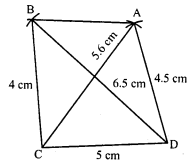(ii) With centre C and radius 5.6 cm and with centre D and radius 4.5 cm, draw arcs which intersect each other at A.